Скачать презентацию Numerical Evaluation of one-loop QCD Amplitudes Benedikt Biedermann

db19f3616b5cdd74f676df7385e46a0d.ppt

• Количество слайдов: 34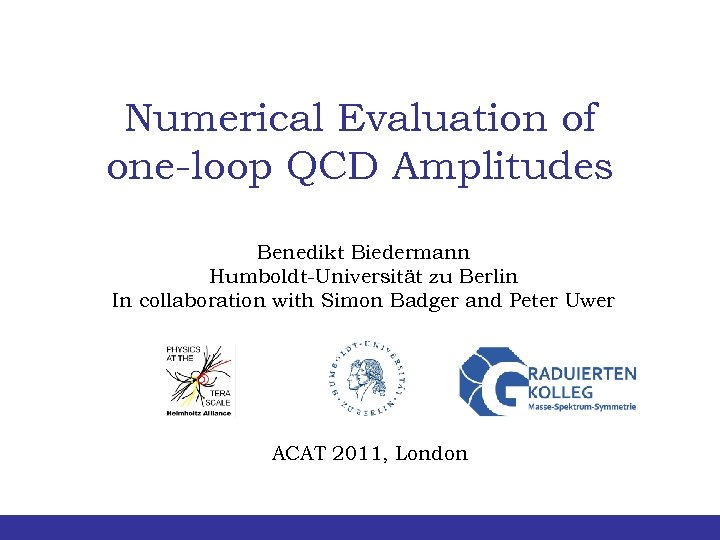Numerical Evaluation of one-loop QCD Amplitudes Benedikt Biedermann Humboldt-Universität zu Berlin In collaboration with Simon Badger and Peter Uwer ACAT 2011, London Benedikt Biedermann | Numerical evaluation of one-loop QCD amplitudes | ACAT 2011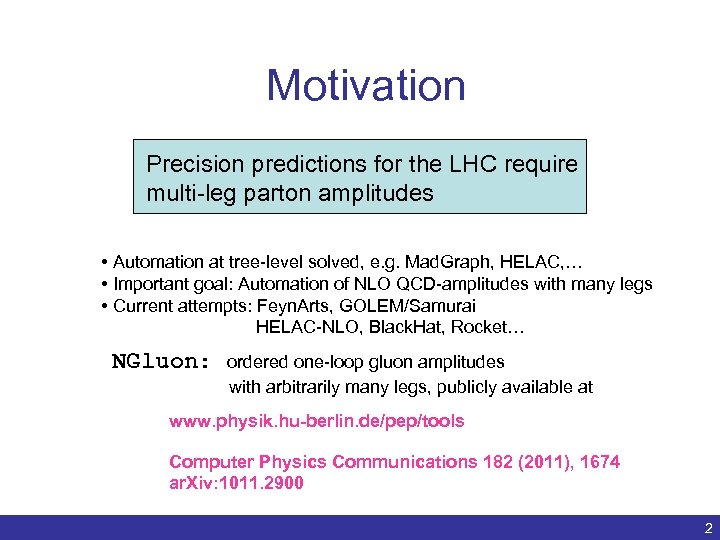Motivation Precision predictions for the LHC require multi-leg parton amplitudes • Automation at tree-level solved, e. g. Mad. Graph, HELAC, … • Important goal: Automation of NLO QCD-amplitudes with many legs • Current attempts: Feyn. Arts, GOLEM/Samurai HELAC-NLO, Black. Hat, Rocket… NGluon: ordered one-loop gluon amplitudes with arbitrarily many legs, publicly available at www. physik. hu-berlin. de/pep/tools Computer Physics Communications 182 (2011), 1674 ar. Xiv: 1011. 2900 Benedikt Biedermann | Numerical evaluation of one-loop QCD amplitudes | ACAT 2011 2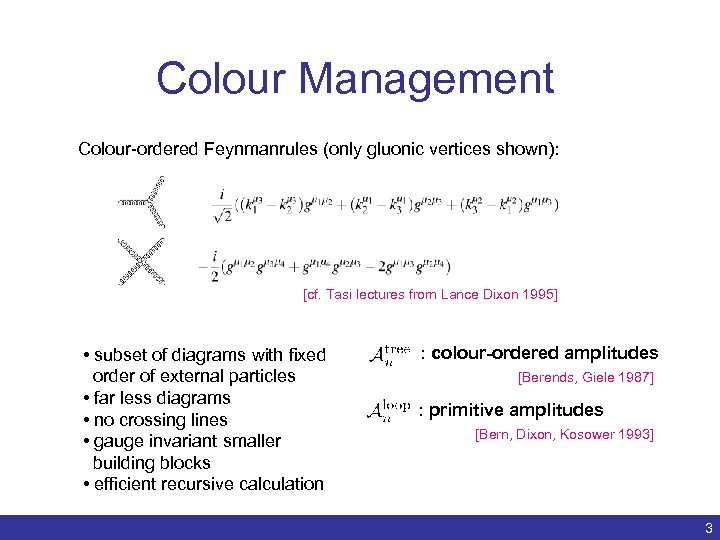Colour Management Colour-ordered Feynmanrules (only gluonic vertices shown): [cf. Tasi lectures from Lance Dixon 1995] • subset of diagrams with fixed order of external particles • far less diagrams • no crossing lines • gauge invariant smaller building blocks • efficient recursive calculation Benedikt Biedermann | : colour-ordered amplitudes [Berends, Giele 1987] : primitive amplitudes [Bern, Dixon, Kosower 1993] Numerical evaluation of one-loop QCD amplitudes | ACAT 2011 3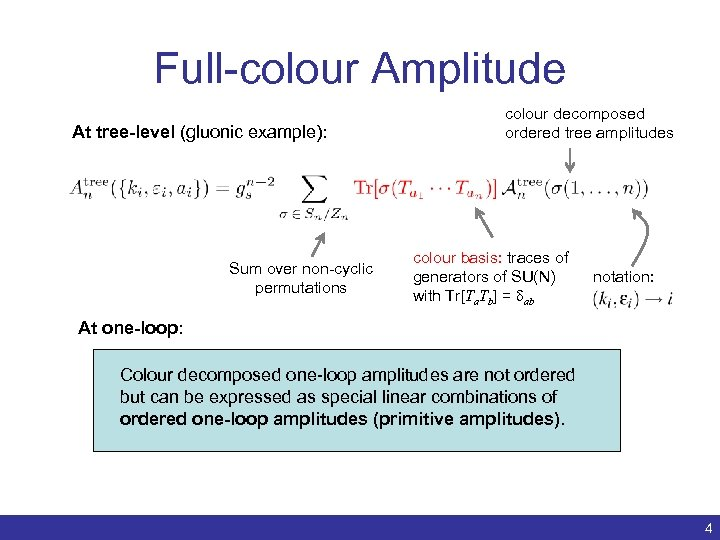Full-colour Amplitude At tree-level (gluonic example): Sum over non-cyclic permutations colour decomposed ordered tree amplitudes colour basis: traces of generators of SU(N) with Tr[Ta. Tb] = dab notation: At one-loop: Colour decomposed one-loop amplitudes are not ordered but can be expressed as special linear combinations of ordered one-loop amplitudes (primitive amplitudes). Benedikt Biedermann | Numerical evaluation of one-loop QCD amplitudes | ACAT 2011 4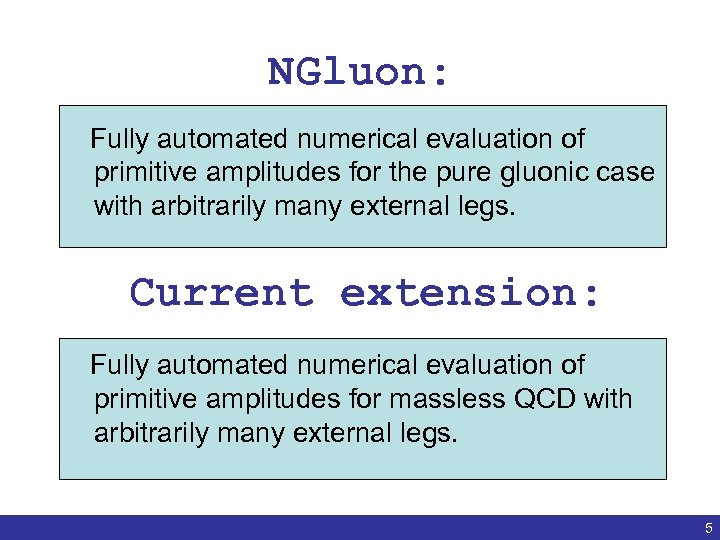NGluon: Fully automated numerical evaluation of primitive amplitudes for the pure gluonic case with arbitrarily many external legs. Current extension: Fully automated numerical evaluation of primitive amplitudes for massless QCD with arbitrarily many external legs. Benedikt Biedermann | Numerical evaluation of one-loop QCD amplitudes | ACAT 2011 5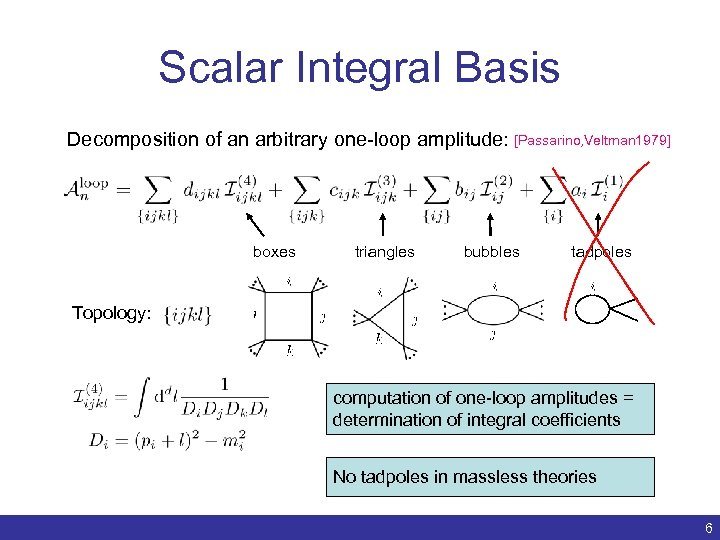Scalar Integral Basis Decomposition of an arbitrary one-loop amplitude: [Passarino, Veltman 1979] boxes triangles bubbles tadpoles Topology: computation of one-loop amplitudes = determination of integral coefficients No tadpoles in massless theories Benedikt Biedermann | Numerical evaluation of one-loop QCD amplitudes | ACAT 2011 6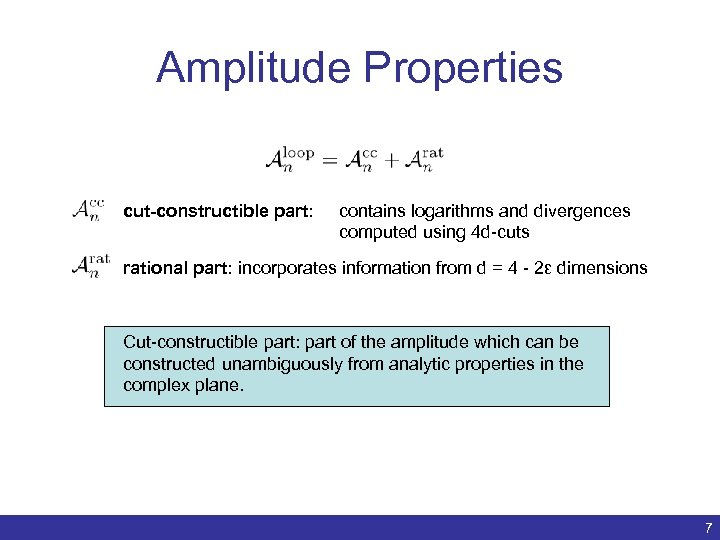Amplitude Properties cut-constructible part: contains logarithms and divergences computed using 4 d-cuts rational part: incorporates information from d = 4 - 2ε dimensions Cut-constructible part: part of the amplitude which can be constructed unambiguously from analytic properties in the complex plane. Benedikt Biedermann | Numerical evaluation of one-loop QCD amplitudes | ACAT 2011 7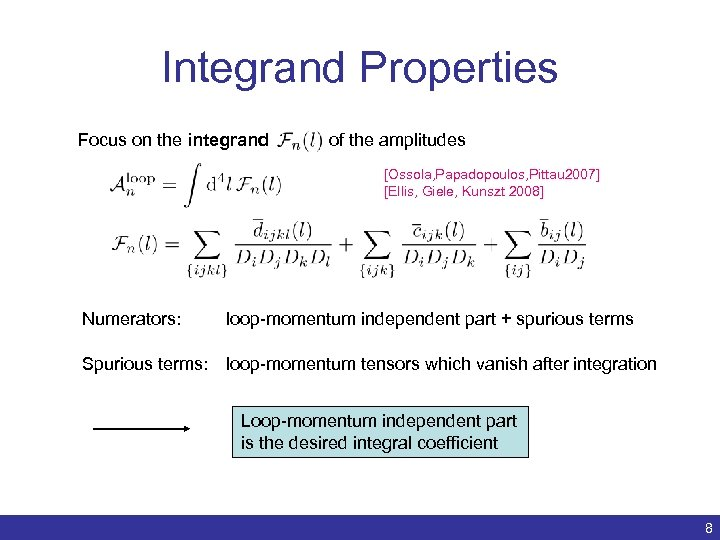Integrand Properties Focus on the integrand of the amplitudes [Ossola, Papadopoulos, Pittau 2007] [Ellis, Giele, Kunszt 2008] Numerators: loop-momentum independent part + spurious terms Spurious terms: loop-momentum tensors which vanish after integration Loop-momentum independent part is the desired integral coefficient Benedikt Biedermann | Numerical evaluation of one-loop QCD amplitudes | ACAT 2011 8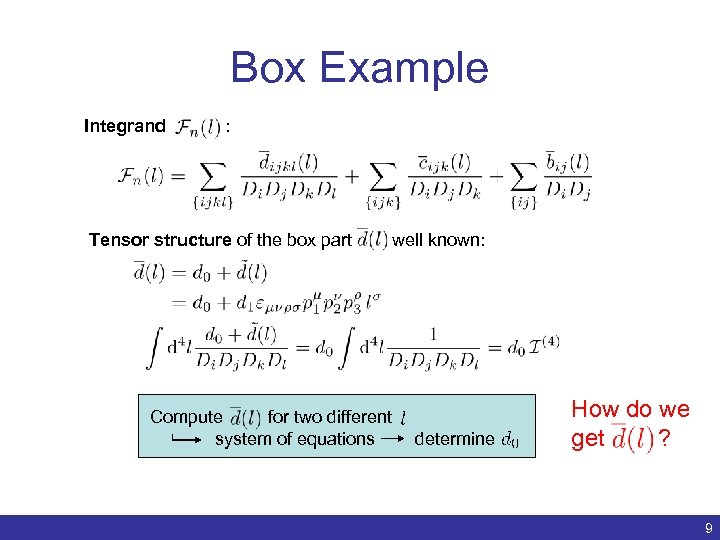Box Example Integrand : Tensor structure of the box part Compute for two different system of equations Benedikt Biedermann | well known: How do we get ? determine Numerical evaluation of one-loop QCD amplitudes | ACAT 2011 9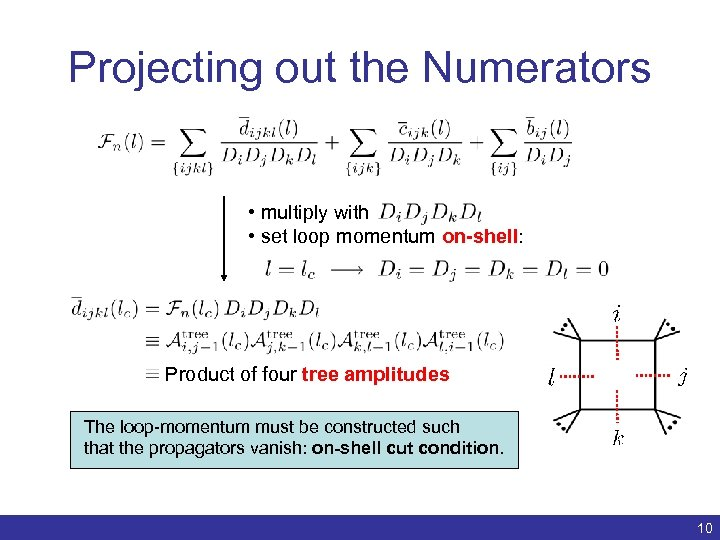Projecting out the Numerators • multiply with • set loop momentum on-shell: Product of four tree amplitudes The loop-momentum must be constructed such that the propagators vanish: on-shell cut condition. Benedikt Biedermann | Numerical evaluation of one-loop QCD amplitudes | ACAT 2011 10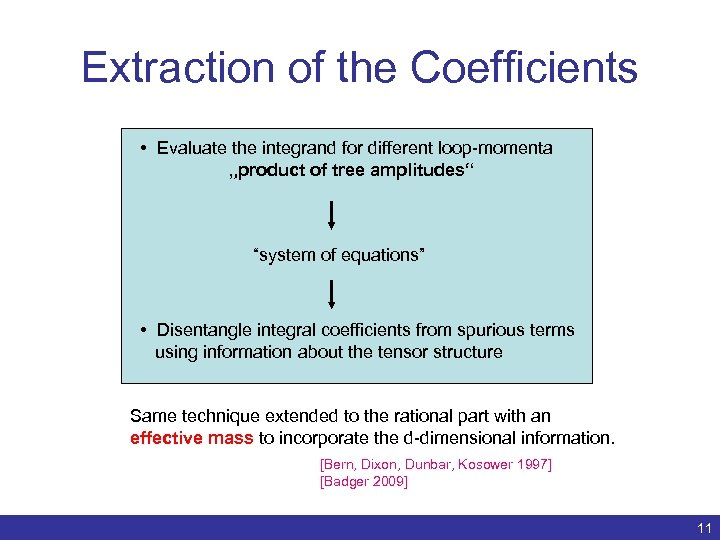Extraction of the Coefficients • Evaluate the integrand for different loop-momenta „product of tree amplitudes“ “system of equations” • Disentangle integral coefficients from spurious terms using information about the tensor structure Same technique extended to the rational part with an effective mass to incorporate the d-dimensional information. [Bern, Dixon, Dunbar, Kosower 1997] [Badger 2009] Benedikt Biedermann | Numerical evaluation of one-loop QCD amplitudes | ACAT 2011 11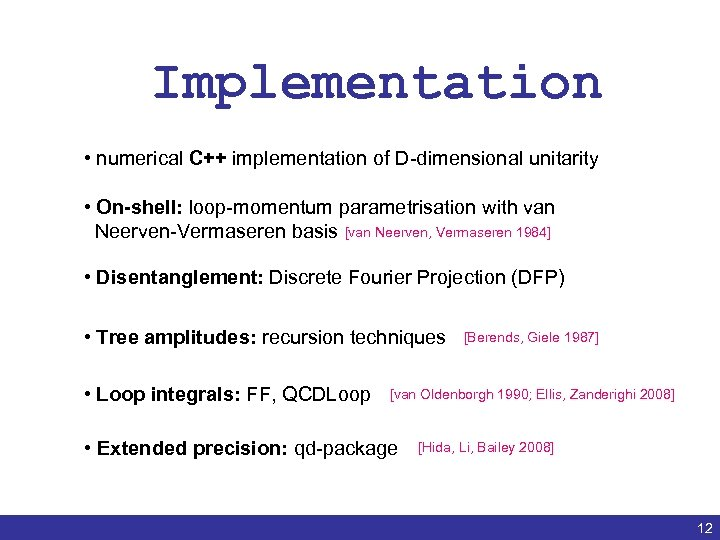Implementation • numerical C++ implementation of D-dimensional unitarity • On-shell: loop-momentum parametrisation with van Neerven-Vermaseren basis [van Neerven, Vermaseren 1984] • Disentanglement: Discrete Fourier Projection (DFP) • Tree amplitudes: recursion techniques • Loop integrals: FF, QCDLoop [van Oldenborgh 1990; Ellis, Zanderighi 2008] • Extended precision: qd-package Benedikt Biedermann | [Berends, Giele 1987] [Hida, Li, Bailey 2008] Numerical evaluation of one-loop QCD amplitudes | ACAT 2011 12Recursive Tree-level Techniques color ordered vertices [Berends, Giele 1987] off shell leg = S + S External wave functions, Polarization vectors Benedikt Biedermann | Numerical evaluation of one-loop QCD amplitudes | ACAT 2011 13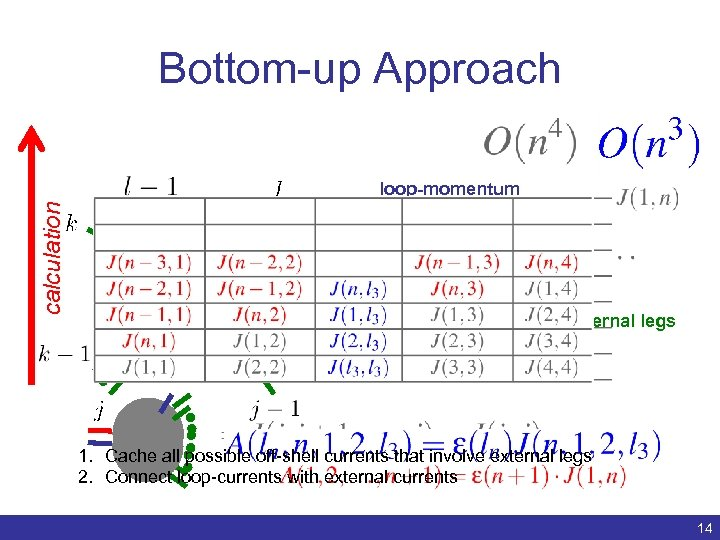Bottom-up Approach calculation loop-momentum 1 parton currents 2 parton currents external legs 3 parton currents 1. Cache all possible off-shell currents that involve external legs 2. Connect loop-currents with external currents Benedikt Biedermann | Numerical evaluation of one-loop QCD amplitudes | ACAT 2011 14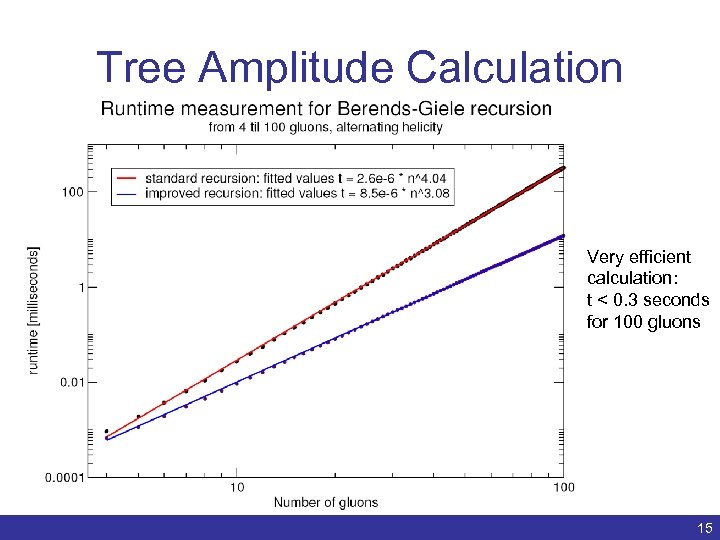Tree Amplitude Calculation Very efficient calculation: t < 0. 3 seconds for 100 gluons Benedikt Biedermann | Numerical evaluation of one-loop QCD amplitudes | ACAT 2011 15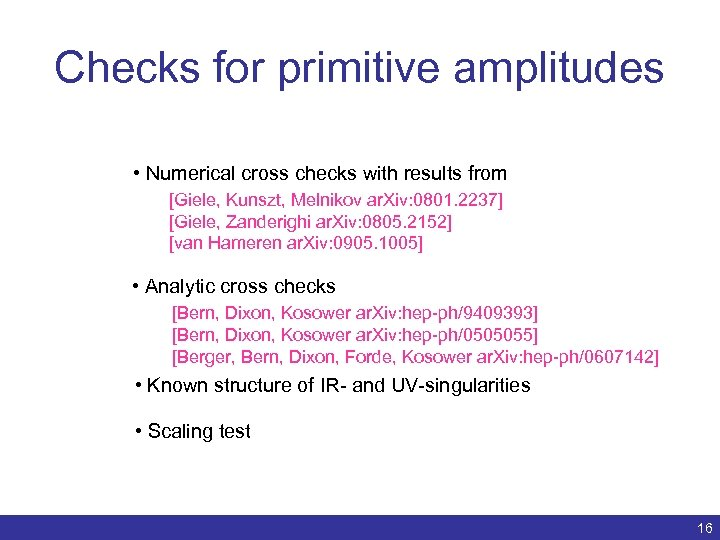Checks for primitive amplitudes • Numerical cross checks with results from [Giele, Kunszt, Melnikov ar. Xiv: 0801. 2237] [Giele, Zanderighi ar. Xiv: 0805. 2152] [van Hameren ar. Xiv: 0905. 1005] • Analytic cross checks [Bern, Dixon, Kosower ar. Xiv: hep-ph/9409393] [Bern, Dixon, Kosower ar. Xiv: hep-ph/0505055] [Berger, Bern, Dixon, Forde, Kosower ar. Xiv: hep-ph/0607142] • Known structure of IR- and UV-singularities • Scaling test Benedikt Biedermann | Numerical evaluation of one-loop QCD amplitudes | ACAT 2011 16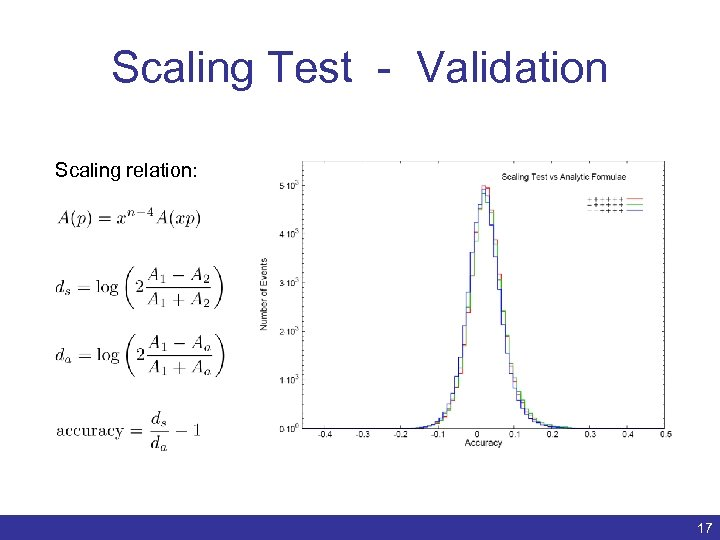Scaling Test - Validation Scaling relation: Benedikt Biedermann | Numerical evaluation of one-loop QCD amplitudes | ACAT 2011 17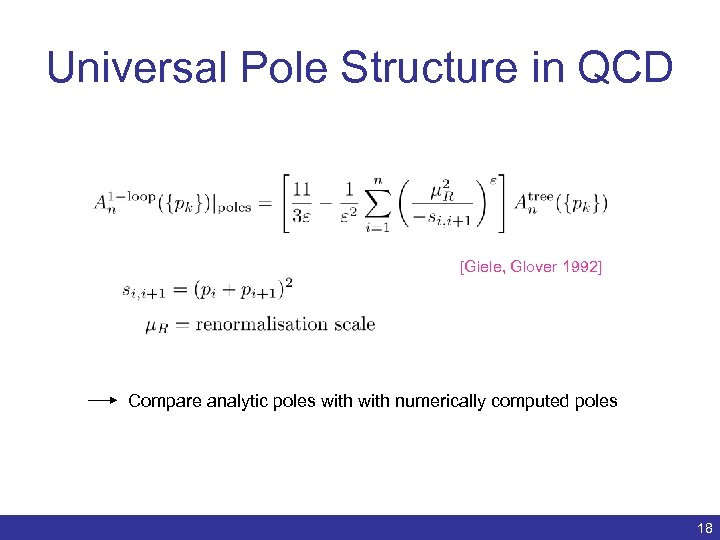Universal Pole Structure in QCD [Giele, Glover 1992] Compare analytic poles with numerically computed poles Benedikt Biedermann | Numerical evaluation of one-loop QCD amplitudes | ACAT 2011 18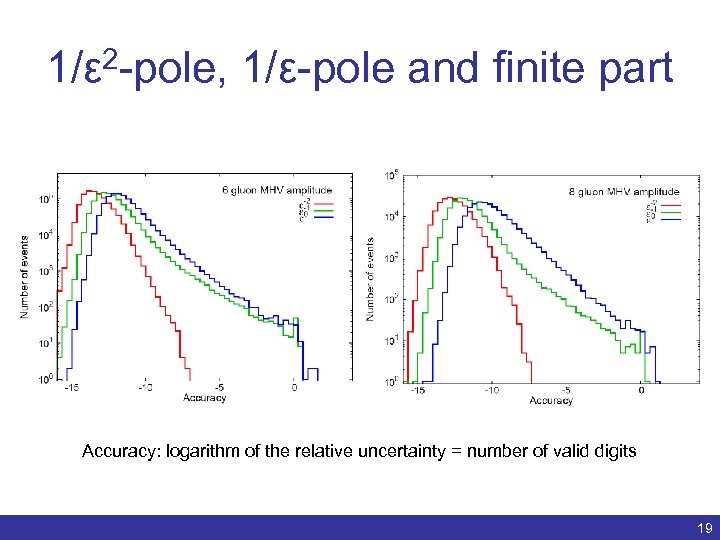1/ε 2 -pole, 1/ε-pole and finite part Accuracy: logarithm of the relative uncertainty = number of valid digits Benedikt Biedermann | Numerical evaluation of one-loop QCD amplitudes | ACAT 2011 19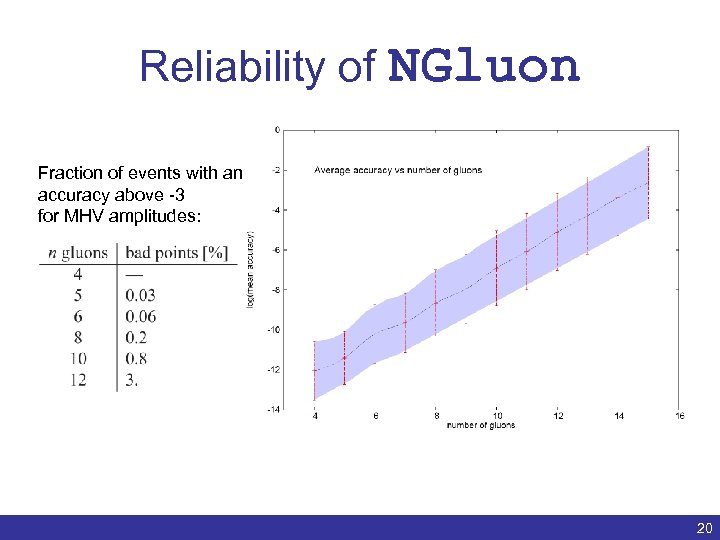Reliability of NGluon Fraction of events with an accuracy above -3 for MHV amplitudes: Benedikt Biedermann | Numerical evaluation of one-loop QCD amplitudes | ACAT 2011 20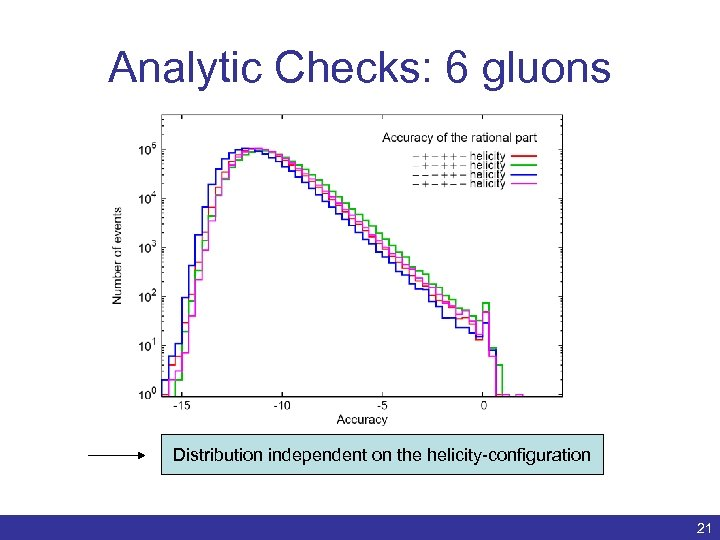Analytic Checks: 6 gluons Distribution independent on the helicity-configuration Benedikt Biedermann | Numerical evaluation of one-loop QCD amplitudes | ACAT 2011 21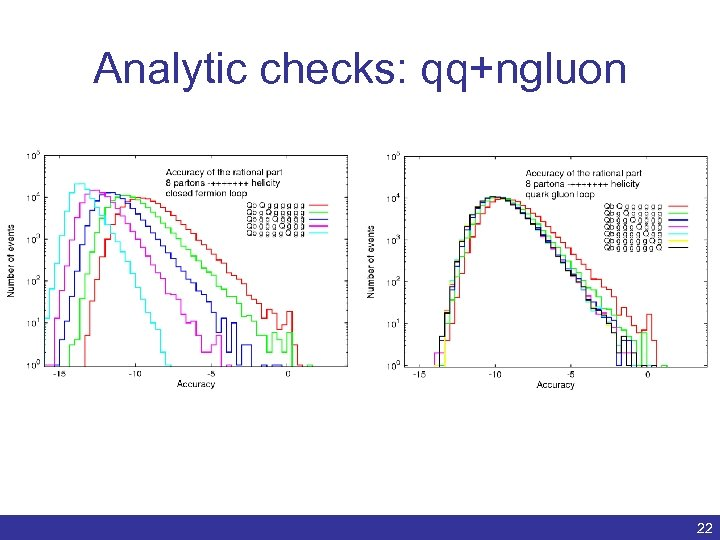Analytic checks: qq+ngluon Benedikt Biedermann | Numerical evaluation of one-loop QCD amplitudes | ACAT 2011 22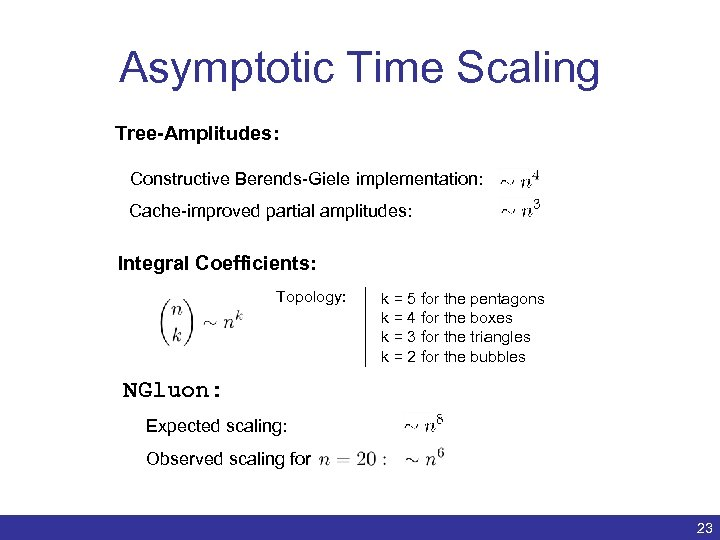Asymptotic Time Scaling Tree-Amplitudes: Constructive Berends-Giele implementation: Cache-improved partial amplitudes: Integral Coefficients: Topology: k = 5 for the pentagons k = 4 for the boxes k = 3 for the triangles k = 2 for the bubbles NGluon: Expected scaling: Observed scaling for Benedikt Biedermann | Numerical evaluation of one-loop QCD amplitudes | ACAT 2011 23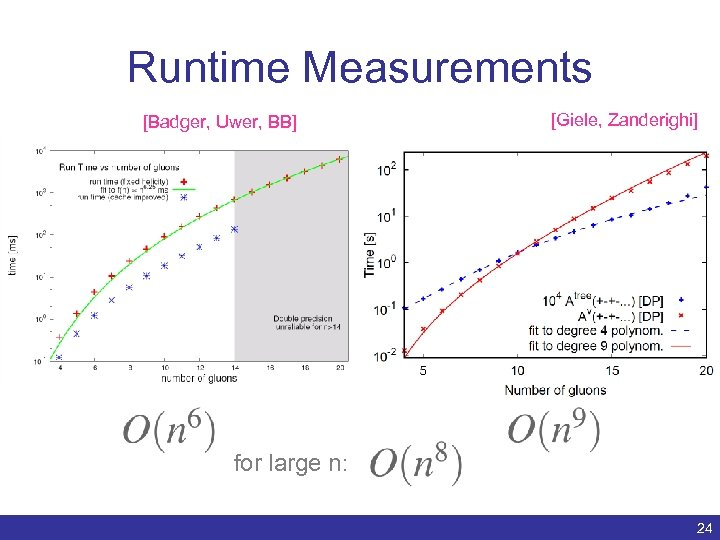Runtime Measurements [Badger, Uwer, BB] [Giele, Zanderighi] for large n: Benedikt Biedermann | Numerical evaluation of one-loop QCD amplitudes | ACAT 2011 24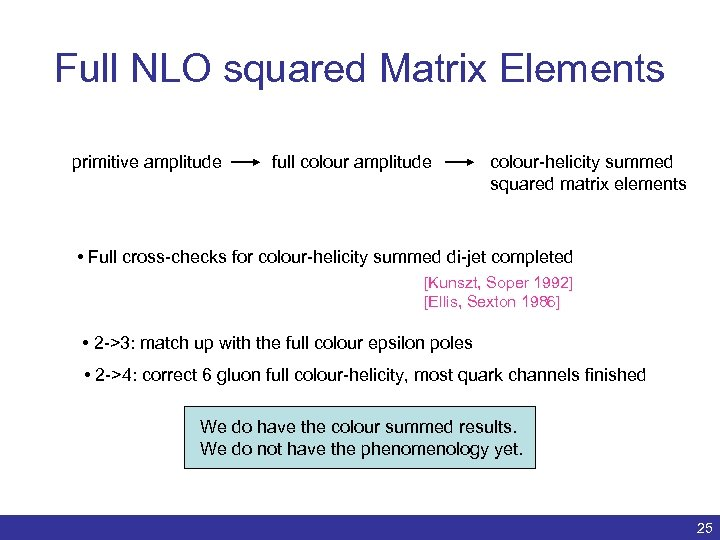Full NLO squared Matrix Elements primitive amplitude full colour amplitude colour-helicity summed squared matrix elements • Full cross-checks for colour-helicity summed di-jet completed [Kunszt, Soper 1992] [Ellis, Sexton 1986] • 2 ->3: match up with the full colour epsilon poles • 2 ->4: correct 6 gluon full colour-helicity, most quark channels finished We do have the colour summed results. We do not have the phenomenology yet. Benedikt Biedermann | Numerical evaluation of one-loop QCD amplitudes | ACAT 2011 25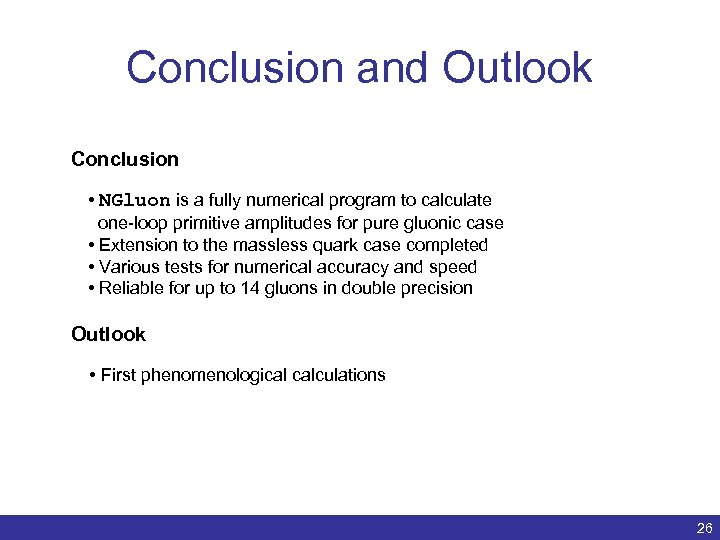Conclusion and Outlook Conclusion • NGluon is a fully numerical program to calculate one-loop primitive amplitudes for pure gluonic case • Extension to the massless quark case completed • Various tests for numerical accuracy and speed • Reliable for up to 14 gluons in double precision Outlook • First phenomenological calculations Benedikt Biedermann | Numerical evaluation of one-loop QCD amplitudes | ACAT 2011 26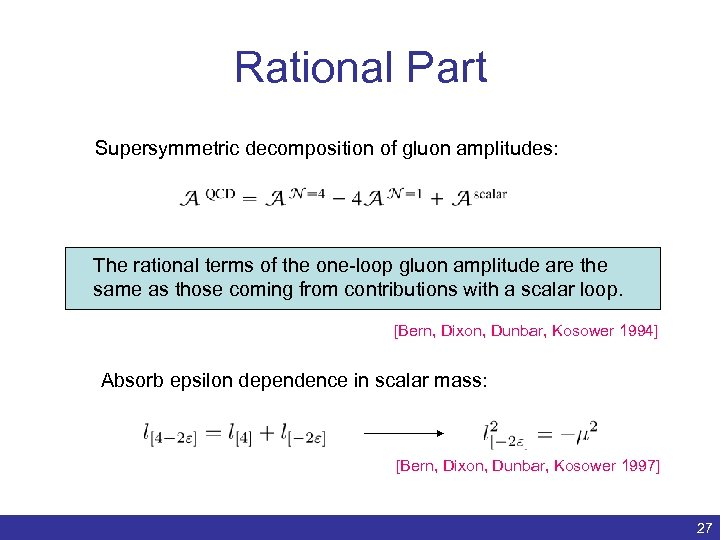Rational Part Supersymmetric decomposition of gluon amplitudes: The rational terms of the one-loop gluon amplitude are the same as those coming from contributions with a scalar loop. [Bern, Dixon, Dunbar, Kosower 1994] Absorb epsilon dependence in scalar mass: [Bern, Dixon, Dunbar, Kosower 1997] Benedikt Biedermann | Numerical evaluation of one-loop QCD amplitudes | ACAT 2011 27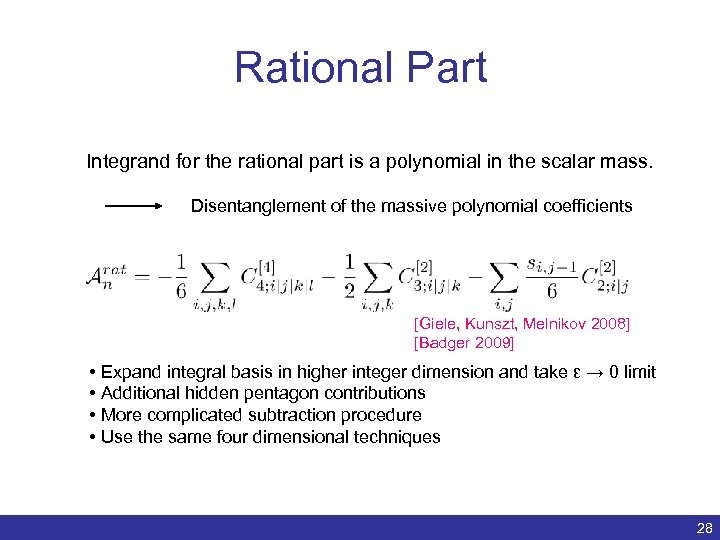Rational Part Integrand for the rational part is a polynomial in the scalar mass. Disentanglement of the massive polynomial coefficients [Giele, Kunszt, Melnikov 2008] [Badger 2009] • Expand integral basis in higher integer dimension and take ε → 0 limit • Additional hidden pentagon contributions • More complicated subtraction procedure • Use the same four dimensional techniques Benedikt Biedermann | Numerical evaluation of one-loop QCD amplitudes | ACAT 2011 28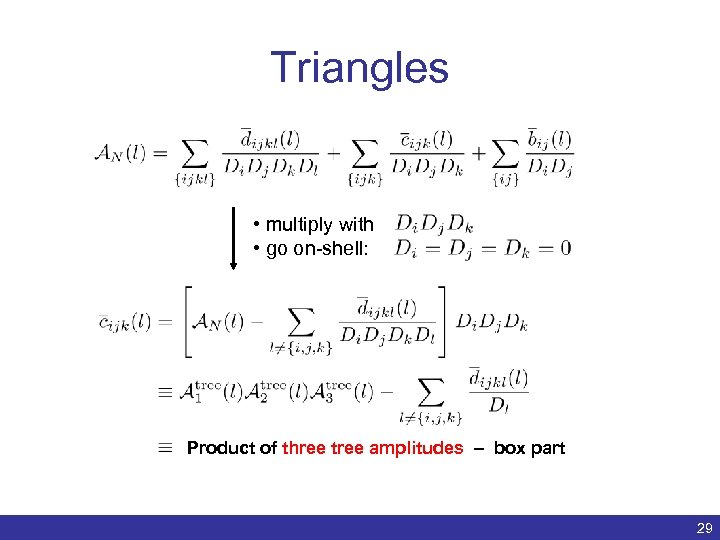Triangles • multiply with • go on-shell: Product of three tree amplitudes – box part Benedikt Biedermann | Numerical evaluation of one-loop QCD amplitudes | ACAT 2011 29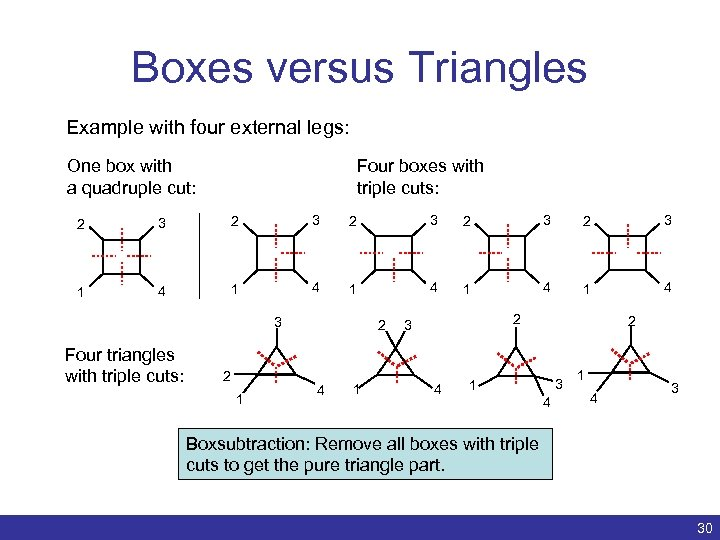Boxes versus Triangles Example with four external legs: One box with a quadruple cut: Four boxes with triple cuts: 2 3 2 3 2 3 1 4 1 4 1 4 3 Four triangles with triple cuts: 2 1 2 4 1 2 3 4 2 3 1 4 3 Boxsubtraction: Remove all boxes with triple cuts to get the pure triangle part. Benedikt Biedermann | Numerical evaluation of one-loop QCD amplitudes | ACAT 2011 30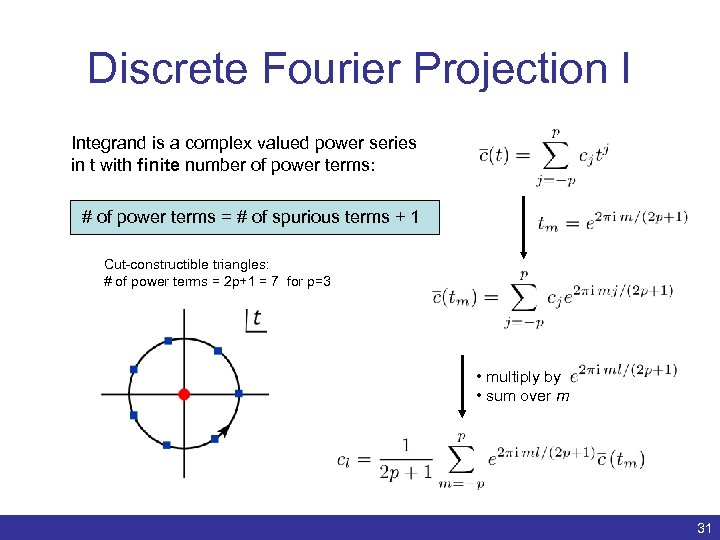Discrete Fourier Projection I Integrand is a complex valued power series in t with finite number of power terms: # of power terms = # of spurious terms + 1 Cut-constructible triangles: # of power terms = 2 p+1 = 7 for p=3 • multiply by • sum over m Benedikt Biedermann | Numerical evaluation of one-loop QCD amplitudes | ACAT 2011 31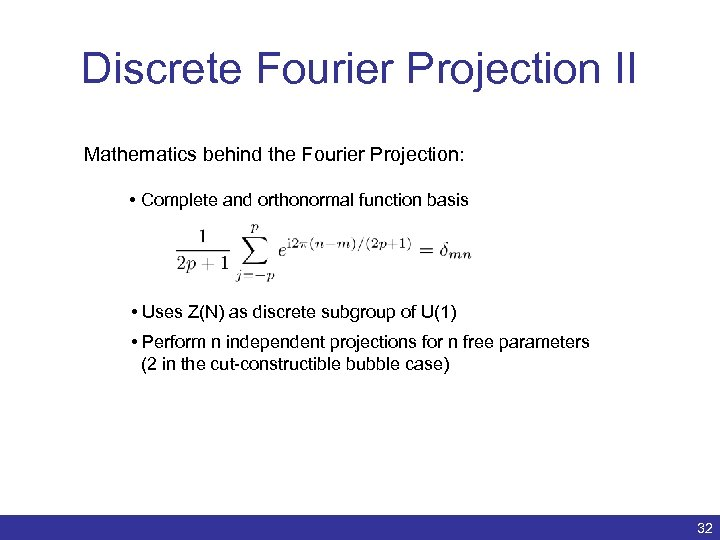Discrete Fourier Projection II Mathematics behind the Fourier Projection: • Complete and orthonormal function basis • Uses Z(N) as discrete subgroup of U(1) • Perform n independent projections for n free parameters (2 in the cut-constructible bubble case) Benedikt Biedermann | Numerical evaluation of one-loop QCD amplitudes | ACAT 2011 32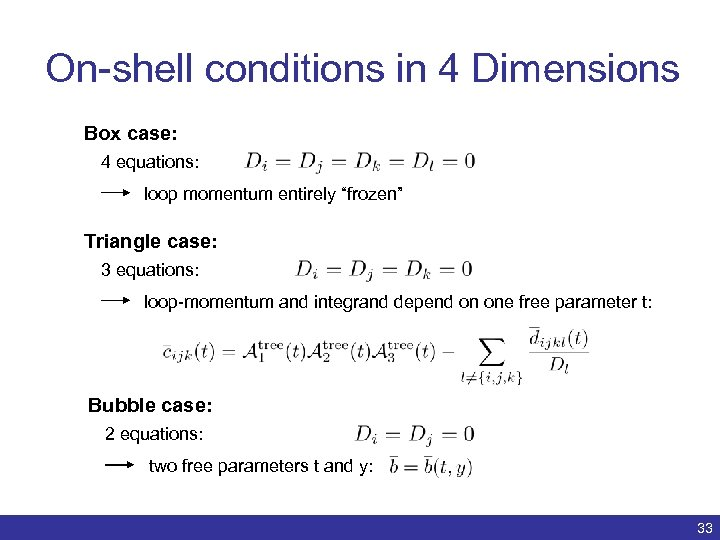On-shell conditions in 4 Dimensions Box case: 4 equations: loop momentum entirely “frozen” Triangle case: 3 equations: loop-momentum and integrand depend on one free parameter t: Bubble case: 2 equations: two free parameters t and y: Benedikt Biedermann | Numerical evaluation of one-loop QCD amplitudes | ACAT 2011 33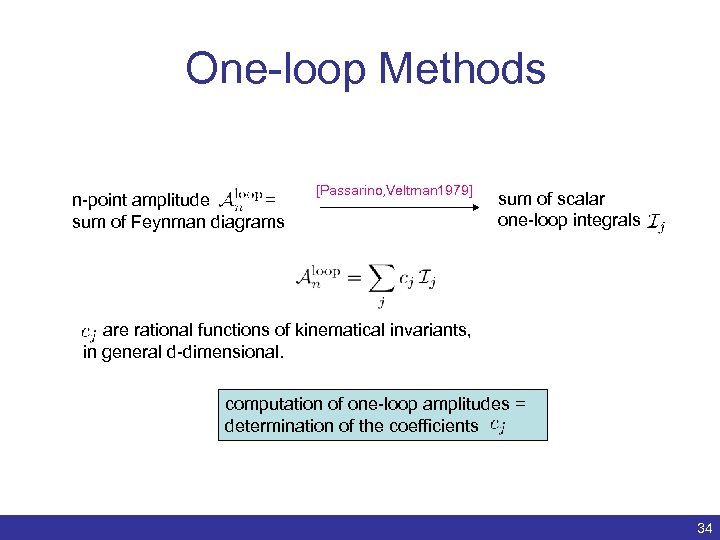One-loop Methods n-point amplitude = sum of Feynman diagrams [Passarino, Veltman 1979] sum of scalar one-loop integrals are rational functions of kinematical invariants, in general d-dimensional. computation of one-loop amplitudes = determination of the coefficients Benedikt Biedermann | Numerical evaluation of one-loop QCD amplitudes | ACAT 2011 34